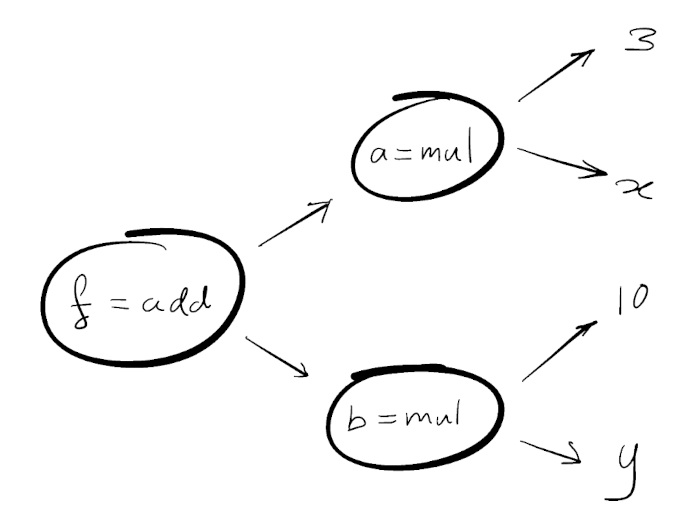## Automatic Differentiation with Python

#### Calculus Lecturers hate this one weird trick

Published 2022/03/24 by Jordan Hay

One of the core concepts of calculus is differentiation, an operation that can take an arbitrary function $f$ and produce a function $f’$ describing the gradient of $f$. Since this can be a repetitive task by hand it would seem to be an ideal job for a computer.

Let’s consider the definition of differentiation (in one dimension)

$$f'(x) = \frac{df}{dx} = \lim\limits_{\Delta x \rightarrow 0} \frac{f(x + \Delta x) - f(x)}{\Delta x}$$

Immediately it can be seen that there is an opening for a method of differentiation where we set $\Delta x$ to be some very small value in order to approximate the derivative of $f$. While this method is effective and a relatively quick task on a computer it quickly leads to inaccurate results, especially for higher order derivatives.

So how could we do better with a computer? A numerical method feels natural given the calculation-oriented nature of computers, but maybe moving closer to how humans produce derivatives (symbolic derivation) will yield better results. In order to produce a symbolic differentiator on a computer we need to decide how to represent the function as something the computer can understand and manipulate.

This function decomposition can be achieved in Python with special objects which track the operations that are performed upon them, building a tree where the root is the final operation, and leaves are variable or constant inputs (Figure 1).Figure 1 - Decomposition tree of the function $f(x, y) = 3x + 10y$.

Analysing this tree as if every node is a function of its own we can see that the chain rule applies. This logical step is the basis of automatic differentiation and the step that allows computers do differentiate symbolically easily.

$$\frac{\partial f}{\partial x} = \frac{df}{da}\cdot\frac{\partial a}{\partial x} + \frac{df}{db}\cdot\frac{db}{dx}$$

It’s worth noting now that this requires the use of the definition of the partial derivative which in two dimensions for a function $f(x, y)$ like above takes the form

$$f_x(x, y) = \frac{\partial f}{\partial x} = \lim\limits_{\Delta x \rightarrow 0} \frac{f(x + \Delta x, y) - f(x, y)}{\Delta x}$$

From here we can define the general form for derivatives of common functions and operations and use the automatic differentiation process to differentiate combinations of these with the chain rule. In jmath

>>> from jmath.autodiff import x, y
>>> f = 3*x + 10*y
>>> f_x = f.d(x)
>>> f_y = f.d(y)
>>>
>>> f_x
3
>>> f_y
10


Here the Variable objects ‘x’ and ‘y’ are used to construct the Function object ‘f’. The differentiate method then applies the automatic differentiation process detailed above, constructing the partial derivatives of the sub-functions per their definition and multiplying and adding them as appropriate. Some jmath universal functions (sin, cos, ln, …)

With some tweaks and the use of the jmath Vector object it is possible to produce gradient vectors and vector valued functions like so

>>> from jmath.autodiff import x, y, z
>>> f = x*y*z
>>> grad = f.d(x, y, z)